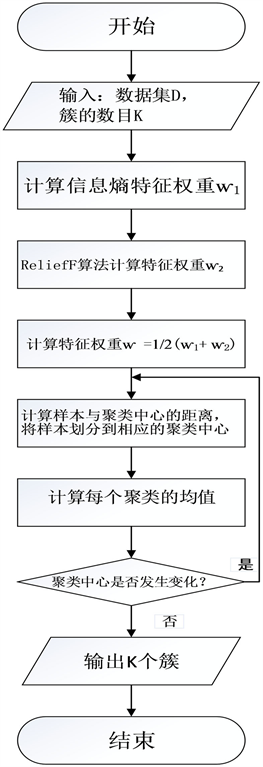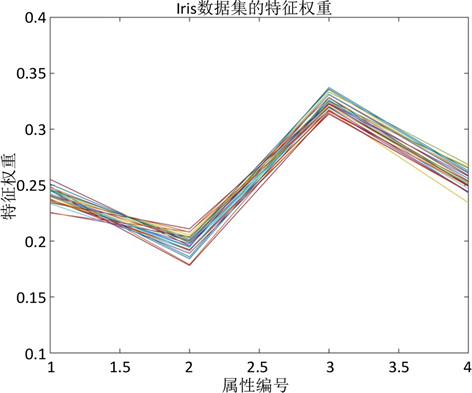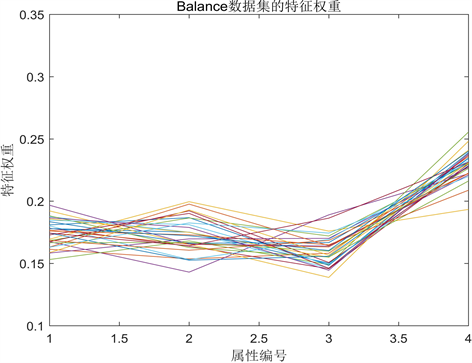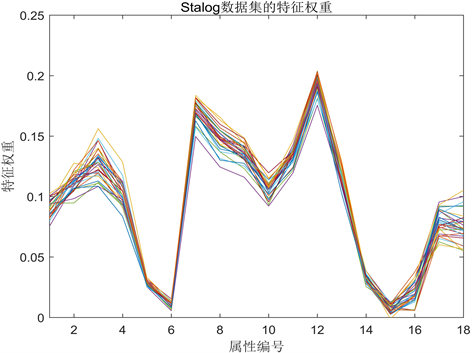﻿ 一种基于特征加权的K-Means算法研究

# 一种基于特征加权的K-Means算法研究A K-Means Algorithm Based on Feature Weighting

Abstract: Cluster analysis is a statistical analysis technique that divides the research objects into relatively homogeneous groups. The core of cluster analysis is to find useful clusters of objects. K-means clustering algorithm has been receiving much attention from scholars because of its excellent speed and good scalability. However, the traditional K-means algorithm does not consider the influence of each attribute on the final clustering result, which makes the accuracy of clustering have a certain impact. In response to the above problems, this thesis proposes an improved feature weighting algorithm. The improved algorithm uses the information entropy and ReliefF feature selection algorithm to weight the features and correct the distance function between clustering objects, so that the algorithm can achieve more accurate and efficient clustering effect. The simulation results show that compared with the traditional K-means algorithm, the improved algorithm clustering results are stable, and the accuracy of clustering is significantly improved.

1. 引言

2. K-means算法

K-means算法的核心思想是通过迭代把数据对象划分到不同的簇中，以求目标函数最小化，从而使生成的簇尽可能的紧凑和独立，算法具体流程如下。

1) 从D中任意选择k个对象作为初始聚类中心；

2) 计算每个对象与这些中心对象的距离；并根据最小距离重新对相应对象进行划分；

3) 重新计算每个聚类的均值；

4) 当满足一定条件，如没有对象再被重新分配给其他的类簇、聚类中心不再发生变化、误差平方和(SSE)最小，则算法终止；如果条件不满足则回到步骤(2)。

$d\left(x,y\right)=\sqrt{{\sum }_{j=1}^{m}{\left({x}_{j}-{y}_{j}\right)}^{2}}$ (1)

3. 基于特征加权的改进算法

3.1. 信息熵

1948年，信息论之父克劳德•艾尔伍德•香农在他发表的论文“通信的数学理论(A Mathematical Theory of Communication)”中，提出了“信息熵”的概念。香农指出，任何信息都存在冗余，冗余大小与信息中每个符号(数字、字母或单词)的出现概率或者说不确定性有关。他用“信息熵”描述信息的不确定性。信息熵的数学表达式如下：

$H\left(x\right)=-{\sum }_{i=1}^{m}{p}_{i}{\mathrm{log}}_{2}{p}_{i}$ (2)

3.2. RliefF算法

1994年，Knonenko提出了ReliefF算法，它是Relief算法的扩展，主要处理多分类问题  。ReliefF算法的基本思想是从训练样本集中随机取出一个样本xi；然后从与xi同类的样本中取出k个近邻样本Hi；再从其他与xi不同的类中分别取出k个样本Mi；根据权重公式更新每个特征的权重；随机抽取m次，得到最终的特征权重。

$\begin{array}{l}w\left(j\right)=w\left(j\right)+{\sum }_{c\ne class\left(i\right)}\frac{\frac{p\left(c\right)}{1-p\left(class\left({x}_{i}\right)\right)}\underset{j=1}{\overset{k}{\sum }}d\left({x}_{i}\left(j\right),{M}_{i}\left(j\right)\right)}{mk}\\ \text{}-\underset{j=1}{\overset{k}{\sum }}\frac{d\left({x}_{i}\left(j\right),{H}_{i}\left(j\right)\right)}{mk}\end{array}$ (3)

$d\left({x}_{i}\left(j\right),{M}_{i}\left(j\right)\right)=|\frac{{x}_{i}\left(j\right)-{M}_{i}\left(j\right)}{\mathrm{max}\left(j\right)-\mathrm{min}\left(j\right)}|$ (4)

ReliefF算法用于处理多分类问题，每个样本都要有明确的类标记。但聚类分析中的样本没有类标记。所以，需要先对样本集进行一次初聚类，获得样本的类标记，再用ReliefF算法进行特征权重的计算。

3.3. 基于特征加权的改进算法ER_Kmeans算法(EntropyReliefF_Kmeans)

ER_Kmeans算法的基本思想是将聚类对象的特征权重与信息熵作为K-means算法的特征权重进行聚类。设信息熵权重为w1，特征权重为w2，则最终的特征权重为

$w=\frac{{w}_{1}+{w}_{2}}{2}$ (5)

ER_Kmeans算法的步骤如下(图1)：Figure 1. ER_Kmeans algorithm flowchart

1) 随机选择K个初始聚类中心；

2) 计算信息熵特征权重ⱳ1；

3) ReliefF算法计算特征权重ⱳ2；

4) 计算特征权重ⱳ；

5) 计算每个样本与这些聚类中心的距离 $\text{d}\left(x,y\right)=\sqrt{\underset{j=1}{\overset{m}{\sum }}{\omega }_{j}{\left({x}_{j}-{y}_{j}\right)}^{2}}$ ；并根据最小距离将样本划分到相应的聚类中心；

6) 重新计算每个聚类的均值；

7) 若聚类中心不再发生变化，则算法终止；如果聚类中心发生变化则回到步骤(5)。

4. 仿真实验

4.1. 实验环境与数据集

4.2. 实验结果与分析Figure 2. Feature weights of the Iris datasetFigure 3. Feature weights for Balance-scale datasetsFigure 4. Feature weights of the Stalog data setTable 2. Comparison of accuracy of each algorithm in UCI dataset (%)Table 3. Comparison of error square sum (SSE) of each algorithm in UCI dataset (/ms2)Table 4. Comparison of the number of iterations of each algorithm in the UCI data set (/times)Table 5. Runtime comparison of each algorithm in UCI dataset (/ms)Table 6. The average run time of each algorithm in the UCI data set is compared with each iteration (/ms)

5. 结束语

 Alexandropoulos, A., Plessas, F. and Birbas, M. (2010) A Dynamic DFI-Compatible Strobe Qualification System for Double Data Rate (DDR) Physical Interfaces. IEEE International Conference on Electronics, Athens, 12-15 December 2010, 277-280. https://doi.org/10.1109/ICECS.2010.5724507

 卓金武, 周英. 量化投资: MATLAB数据挖掘技术与实践[M]. 北京: 电子工业出版社, 2017: 217-224.

 刘铭, 吴冲. 基于特征权重量化的相似度计算方法[J]. 计算机学报, 2015, 38(7): 1420-1433.

 Li, J. and Gao, X.B. (2006) A New Feature Weighted Fuzzy Clustering Algorithm. Proceedings of SPIE, The Inter-national Society for Optical Engineering, 3641, 412-420.

 Meng, Q. (2015) An Improved Clustering Algorithm Based on Fea-ture-Weight Learning. Journal of Information and Computational Science, 12, 3519-3526.
https://doi.org/10.12733/jics20106074

 Shang, S.T. and Shi, M.Y. (2016) Improved Feature Weight Algorithm and Its Ap-plication to Text Classification. Mathematical Problems in Engineering, 2016, Article ID: 7819626.
https://doi.org/10.1155/2016/7819626

 杨玉梅. 基于信息熵改进的K-means动态聚类算法[J]. 重庆邮电大学学报(自然科学版), 2016, 28(2): 254-259.

 菅小艳, 韩素青. 不平衡数据集上的Relief特征选择算法[J]. 数据采集与处理, 2016, 31(4): 838-844.

 UCI Machine Learning Repository: Data Sets [DB]. http://archive.ics.uci.edu/ml/datasets.html

Top